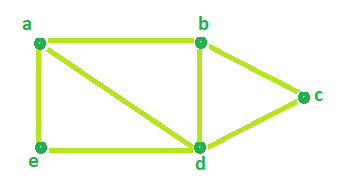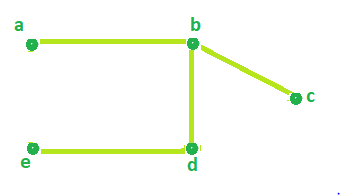Program to find Circuit Rank of an Undirected Graph

• Difficulty Level : Basic
• Last Updated : 14 Apr, 2021

Given the number of Vertices and the number of Edges of an Undirected Graph. The task is to determine the Circuit rank.
Circuit Rank: The Circuit rank of an undirected graph is defined as the minimum number of edges that must be removed from the graph to break all of its cycles, converting it into a tree or forest.
Examples

Input : Edges = 7 , Vertices = 5
Output : Circuit rank = 3

Input : Edges = 7 , Vertices = 6
Output : Circuit rank = 2

Formula

Circuit rank = Edges - (Vertices - 1)

Look at the sample graph below,Total number of Edges = 7 and Vertices = 5.
According to the above formula,

Circuit Rank =  Edges - (Vertices - 1)
=  7 - (5 - 1)
= 3

Therefore, Circuit Rank of the above graph = 3.
It can be seen in the below image that by removing 3 edges(a-d, a-e, c-d) from the above graph, all of it cycles can be removed.Below is the implementation of the above approach:

C++

 // C++ Program to find Circuit Rank of an Undirected Graph #include using namespace std;   // Function that calculates the // Circuit rank of the Graph. int Rank(int Edges, int Vertices) {     int result = 0;       // calculates Circuit Rank     result = Edges - Vertices + 1;       return result; }   // Driver Code int main() {     int Edges = 7, Vertices = 5;       cout << "Circuit Rank = " << Rank(Edges, Vertices);       return 0; }

Java

 //Java Program to find Circuit Rank of an Undirected Graph   public class GFG {       //Function that calculates the     //Circuit rank of the Graph.     static int Rank(int Edges, int Vertices)     {      int result = 0;        // calculates Circuit Rank      result = Edges - Vertices + 1;        return result;     }       //Driver Code     public static void main(String[] args) {                    int Edges = 7, Vertices = 5;            System.out.println("Circuit Rank = " + Rank(Edges, Vertices));     } }

Python 3

 # Python 3 program to find Circuit Rank of # an Undirected Graph   # Function that calculates the # Circuit rank of the Graph. def Rank(Edges, Vertices) :       # calculates Circuit Rank     result = Edges - Vertices + 1       return result   # Driver code     if __name__ == "__main__" :       Edges, Vertices = 7, 5       print("Circuit Rank =",Rank(Edges, Vertices))     # This code is contributed by ANKITRAI1

C#

 // C# Program to find Circuit // Rank of an Undirected Graph using System;   class GFG {   // Function that calculates the // Circuit rank of the Graph. static int Rank(int Edges,                 int Vertices) {     int result = 0;           // calculates Circuit Rank     result = Edges - Vertices + 1;           return result; }   // Driver Code public static void Main() {     int Edges = 7, Vertices = 5;       Console.WriteLine("Circuit Rank = " +                        Rank(Edges, Vertices)); } }   // This code is contributed // by inder_verma



Javascript



Output:

Circuit Rank = 3

My Personal Notes arrow_drop_up
Recommended Articles
Page :